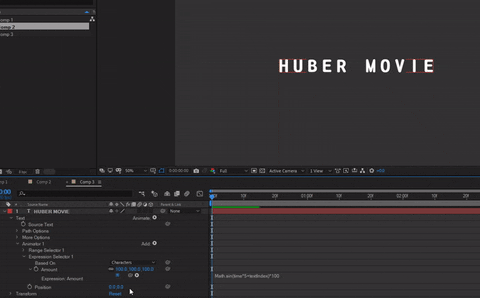### 《AE教學》利用Math.sin製作Wave效果！Math.sin表達式進階運用！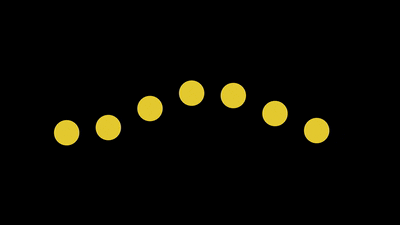## 1.Math.sin簡介x = ;

y = ;

value+[x,y]time*5代表頻率為5，## 2.一般圖形Wave效果y定義為Math.sin(time*5+index)*100，

x = index*200;
y = Math.sin(time*5+index)*100;
value+[x,y]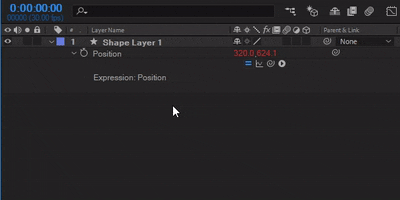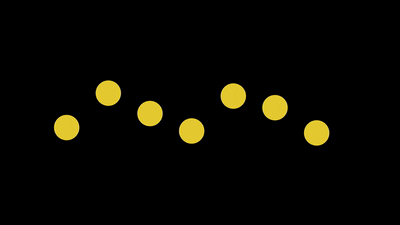## 3.文字Wave效果Position就會被移至Expression底下囉！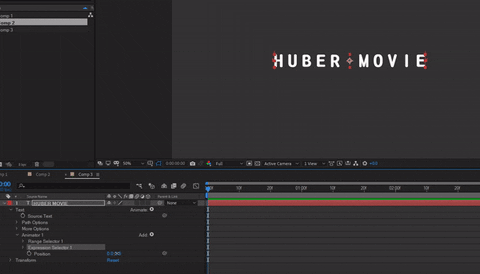Math.sin(time*5+textIndex)*100，